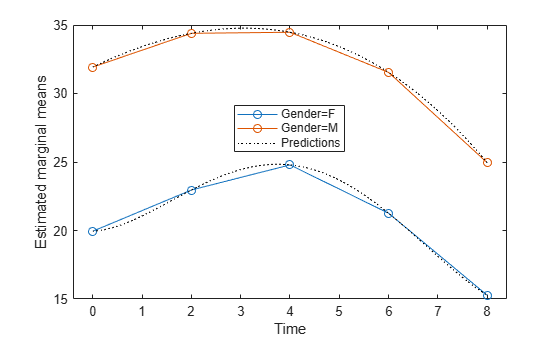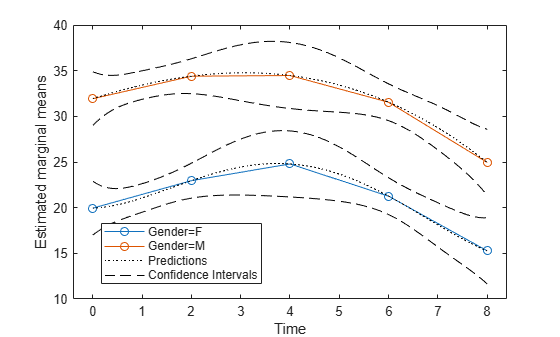predict

Class: RepeatedMeasuresModel

Compute predicted values given predictor values

Description

example

ypred = predict(rm,tnew) returns the predicted values from the repeated measures model rm using the predictor values from the table t.

example

ypred = predict(rm,tnew,Name,Value) returns the predicted values from the repeated measures model rm with additional options specified by one or more Name,Value pair arguments.

For example, you can specify the within-subjects design matrix.

example

[ypred,yci] = predict(___) also returns the 95% confidence interval for the predicted values.

Input Arguments

expand all

Repeated measures model, returned as a RepeatedMeasuresModel object.

For properties and methods of this object, see RepeatedMeasuresModel.

New data including the values of the response variables and the between-subject factors used as predictors in the repeated measures model, rm, specified as a table. tnew must contain all of the between-subject factors used to create rm.

Name-Value Arguments

Specify optional comma-separated pairs of Name,Value arguments. Name is the argument name and Value is the corresponding value. Name must appear inside quotes. You can specify several name and value pair arguments in any order as Name1,Value1,...,NameN,ValueN.

Significance level of the confidence intervals for the predicted values, specified as the comma-separated pair consisting of 'alpha' and a scalar value in the range of 0 to 1. The confidence level is 100*(1–alpha)%.

Example: 'alpha',0.01

Data Types: double | single

Model for the within-subject factors, specified as the comma-separated pair consisting of 'WithinModel' and one of the following:

• 'separatemeans' — Compute a separate mean for each group.

• 'orthogonalcontrasts' — Valid when the within-subject design consists of a single numeric factor T. This specifies a model consisting of orthogonal polynomials up to order T(r-1), where r is the number of repeated measures.

• A character vector or string scalar that defines a model specification in the within-subject factors.

Example: 'WithinModel','orthogonalcontrasts'

Data Types: char | string

Design for within-subject factors, specified as the comma-separated pair consisting of 'WithinDesign' and a vector, matrix, or a table. It provides the values of the within-subject factors in the same form as the RM.WithinDesign property.

Example: 'WithinDesign','Time'

Data Types: single | double | table

Output Arguments

expand all

Predicted values from the repeated measures model rm, returned as an n-by-r matrix, where n is the number of rows in tnew and r is the number of repeated measures in rm.

Confidence intervals for predicted values from the repeated measures model rm, returned as an n-by-r-by-2 matrix.

These are nonsimultaneous intervals for predicting the mean response at the specified predictor values. For predicted value ypred(i,j), the lower limit of the interval is yci(i,j,1) and the upper limit is yci(i,j,2).

Examples

expand all

The column vector, species consists of iris flowers of three different species: setosa, versicolor, and virginica. The double matrix meas consists of four types of measurements on the flowers: the length and width of sepals and petals in centimeters, respectively.

Store the data in a table array.

t = table(species,meas(:,1),meas(:,2),meas(:,3),meas(:,4), ...
'VariableNames',{'species','meas1','meas2','meas3','meas4'});
Meas = dataset([1 2 3 4]','VarNames',{'Measurements'});

Fit a repeated measures model, where the measurements are the responses and the species is the predictor variable.

rm = fitrm(t,'meas1-meas4~species','WithinDesign',Meas);

Predict responses for the three species.

Y = predict(rm,t([1 51 101],:))
Y = 3×4

5.0060    3.4280    1.4620    0.2460
5.9360    2.7700    4.2600    1.3260
6.5880    2.9740    5.5520    2.0260

The matrix Y contains response data for 16 individuals. The response is the blood level of a drug measured at five time points (time = 0, 2, 4, 6, and 8). Each row of Y corresponds to an individual, and each column corresponds to a time point. The first eight subjects are female, and the second eight subjects are male. This is simulated data.

Define a variable that stores gender information.

Gender = ['F' 'F' 'F' 'F' 'F' 'F' 'F' 'F' 'M' 'M' 'M' 'M' 'M' 'M' 'M' 'M']';

Store the data in a proper table array format to perform repeated measures analysis.

t = table(Gender,Y(:,1),Y(:,2),Y(:,3),Y(:,4),Y(:,5), ...
'VariableNames',{'Gender','t0','t2','t4','t6','t8'});

Define the within-subjects variable.

Time = [0 2 4 6 8]';

Fit a repeated measures model, where the blood levels are the responses and gender is the predictor variable.

rm = fitrm(t,'t0-t8 ~ Gender','WithinDesign',Time);

Predict the responses at intermediate times.

time = linspace(0,8)';
Y = predict(rm,t([1 5 8 12],:), ...
'WithinModel','orthogonalcontrasts','WithinDesign',time);

Plot the predictions along with the estimated marginal means.

plotprofile(rm,'Time','Group',{'Gender'})
hold on;
plot(time,Y,'Color','k','LineStyle',':');
legend('Gender=F','Gender=M','Predictions')
hold offThe matrix Y contains response data for 16 individuals. The response is the blood level of a drug measured at five time points (time = 0, 2, 4, 6, and 8). Each row of Y corresponds to an individual, and each column corresponds to a time point. The first eight subjects are female, and the second eight subjects are male. This is simulated data.

Define a variable that stores gender information.

Gender = ['F' 'F' 'F' 'F' 'F' 'F' 'F' 'F' 'M' 'M' 'M' 'M' 'M' 'M' 'M' 'M']';

Store the data in a proper table array format to perform repeated measures analysis.

t = table(Gender,Y(:,1),Y(:,2),Y(:,3),Y(:,4),Y(:,5), ...
'VariableNames',{'Gender','t0','t2','t4','t6','t8'});

Define the within-subjects variable.

Time = [0 2 4 6 8]';

Fit a repeated measures model, where the blood levels are the responses and gender is the predictor variable.

rm = fitrm(t,'t0-t8 ~ Gender','WithinDesign',Time);

Predict the responses at intermediate times.

time = linspace(0,8)';
[ypred,ypredci] = predict(rm,t([1 5 8 12],:), ...
'WithinModel','orthogonalcontrasts','WithinDesign',time);

Plot the predictions and the confidence intervals for predictions along with the estimated marginal means.

p1 = plotprofile(rm,'Time','Group',{'Gender'});
hold on;
p2 = plot(time,ypred,'Color','k','LineStyle',':');
p3 = plot(time,ypredci(:,:,1),'k--');
p4 = plot(time,ypredci(:,:,2),'k--');
legend([p1;p2(1);p3(1)],'Gender=F','Gender=M','Predictions','Confidence Intervals')
hold off